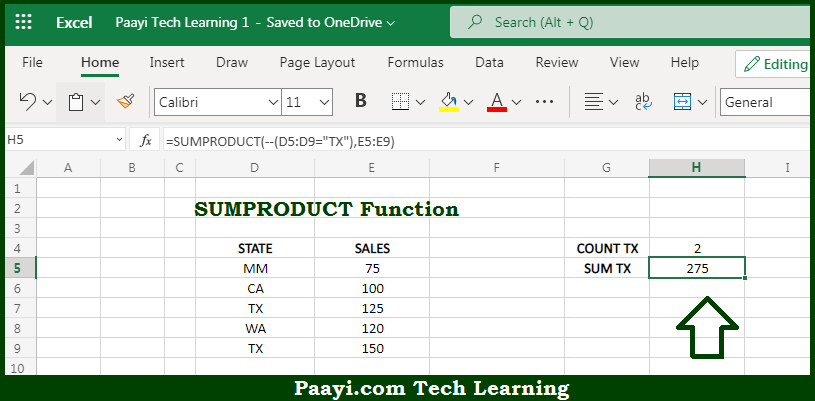# Learn How to Use Microsoft Excel SUMPRODUCT Function

Written by | 0 Comments | 552 Views

In this article, you will learn how to use the Microsoft Excel SUMPRODUCT function and its prime function in Microsoft Excel. You will also get to know the Microsoft Excel SUMPRODUCT function return value and syntax with the help of some examples.

Microsoft Excel SUMPRODUCT Function

The main function of the Microsoft Excel SUMPRODUCT function is to multiply and then sum arrays. That implies, with the help of the SUMPRODUCT function you can able to multiply ranges or arrays together and returns the sum of products. This may sound weird, but the SUMPRODUCT function is a very versatile function that can be used to count and sum like COUNTIFS or SUMIFS, and provide more flexibility. Other functions can easily be used inside SUMPRODUCT functions to improve functionality even further. So, with the help of the SUMPRODUCT function, you can able to multiply and then sum arrays.

Return Value of SUMPRODUCT Function

The return value will be the multiplied and summed arrays.

Syntax of SUMPRODUCT Function

=SUMPRODUCT(array1, [array2], ...)

Where the arguments:

• array1: This is the first range or array that needs to multiply and then add.
• array2: This is the second range or array that needs to be multiplied and then add (optional).

## How to Use Microsoft Excel SUMPRODUCT Function?So we know that Microsoft Excel SUMPRODUCT function you can able to multiply and then sum arrays. That implies, with the help of the SUMPRODUCT function you can able to multiply ranges or arrays together and returns the sum of products. This may sound weird, but the SUMPRODUCT function is a very versatile function that can be used to count and sum like COUNTIFS or SUMIFS, and provide more flexibility.

Other functions can easily be used inside SUMPRODUCT functions to improve functionality even further. So, with the help of the SUMPRODUCT function, you can able to multiply and then sum arrays.